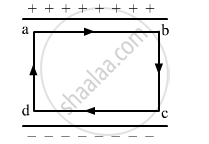# Find the Amount of Work Done in Moving a Charge Q Over a Closed Loop a B C D A. - Physics

The electric field inside a parallel plate capacitor is E. Find the amount of work done in moving a charge q over a closed loop a b c d a.#### Solution

Work done, W= F.d

Here, F is the force exerted on the charge (q) due to electric field (E) and is given by:

F=qE

Net displacement, d = 0

W=0

Concept: Combination of Capacitors
Is there an error in this question or solution?